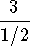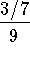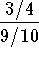#Complex FractionsA complex fraction is a fraction where the numerator, denominator, or both contain a fraction.• Example 1:is a complex fraction. The numerator is 3 and the denominator is 1/2.
• Example 2:is a complex fraction. The numerator is 3/7 and the denominator is 9.
• Example 3:is a complex fraction. The numerator is 3/4 and the denominator is 9/10.

Rule 24: To manipulate complex fractions, just convert them to simple fractions and follow rules 1 through 23 for simple fractions.

If you would like to review another examples of Rule 24 and work problems click on Rule 24.

Rule 25: To multiply two complex fractions, convert the fractions to simple fractions and follow the steps you use to multiply two simple fractions.

If you would like to review another examples of Rule 25 and work problems click on Rule 25.

Rule 26: To multiply add or subtract two complex fractions, convert the fractions to simple fractions and follow the steps you use to add or subtract two simple fractions.

If you would like to review another examples of Rule 26 and work problems click on Rule 26.[Simple Fractions] [Complex Fractions] [Compound Fractions]
[Decimals] [Percentage] [Rules]

[Algebra] [Trigonometry] [Complex Variables]S.O.S MATHematics home page

Do you need more help? Please post your question on our S.O.S. Mathematics CyberBoard.Author: Nancy Marcus